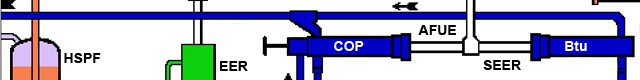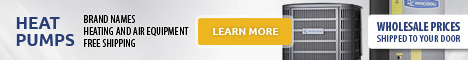# Heat Pump Calculator## Energy and Cost Calculations – Heat Pumps

Important!

Using these energy calculators provides rough figures. You should use the results to get a rough estimate of the size and cost of your required system. To get more accurate information it is advised that you consult an accredited renewable energy supplier.For information about how heat pumps work, see the Heat Pumps article this web site.

Also, be sure to stay on top of your country’s renewable energy subsidy programs as this will make a very big difference to the ultimate costs (see Subsidy Programs this web site).

### Heat Pump Calculators

The objective of using a heat pump calculator is to determine the size, cost and eventual cost savings of the heat pump system you will need based on your heating requirements. These calculators offer various variables and it is a good idea to try them all to get a good idea of what is involved in calculating heat pump requirements. The list of terms below gives an explanation for the variables.

Heat Pump Calculator 1

Heat Pump Calculator 2

Terms

Btu

Btu stands for – British thermal unit – .

1 Btu = 1 055.05585 joules

1 Btu = 0.00029308 kW (kilowatt)

1 kW = 3412 Btu

Btu is a unit of energy used to measure heating and cooling systems, as such, a heating or cooling system will have a Btu rating, e.g., 10,000 Btu.

EER

EER stands for – Energy Efficiency Ratio – . This is the (Btu/hr)/(Watts/hr) of a system at a given operating point. This operating point considers a condition for an indoor temperature and humidity level and an outdoor temperature and humidity level at a given location.

SEER

SEER stands for – Seasonal Energy Efficiency Ratio -. This is the (Btu/hr)/(Watts/hr) of a system at its expected overall performance for a typical year’s weather (temperature and humidity) at a given location. It is generally used for determining the cooling efficiency.

COP

COP stands for – Coefficient of Performance -. This is the measure of the energy efficiency of a system’s ability to change the thermal conditions of an area, based on the ratio of energy input relative to energy output. COP is expressed as:

COP = Thermal Output (Btu/hr) / ((Power Input (kW) * (3413 Btu/kWh))

or

COP = (The change in the heat level of an area) / (The energy consumed)

Because the energy consumed will always generate an amount of heat, calculating the COP for heating and cooling is different, i.e., when calculating the COP for heating efficiency, the heat generated from the energy consumed is added to the change in heat, whereas when calculating for cooling efficiency, the heat generated from the energy consumed is not included. This difference has to do with the system setup, where a cooling system is usually designed to release heat generated from the energy consumed outside of the area to be cooled.

The higher the COP, the more efficient the system.

IPLV

IPLV stands for – Integrated Part Load Value -. The IPLV measures the efficiency of air conditioners under a variety of conditions, these being when the unit is operating at 25%, 50%, 75% and 100% of capacity and at different temperatures. This measurement is mainly for industrial purposes.

AFUE

AFUE stands for – Annual Fuel Utilization Efficiency -. This measures the amount of heat actually delivered to a house compared to the amount of fuel that must be supplied to the furnace, e.g., if the furnace converts 80% of the supplied fuel to heat, then it has an AFUE of 80%. The other 20% is lost to the chimney.

HSPF

HSPF stands for – Heating Seasonal Performance Factor -. This is the (Btu/hr)/(Watts/hr) of a system at its expected overall performance for a typical year’s weather (temperature and humidity) at a given location. It is generally used for determining the heating efficiency.